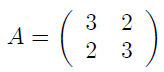## Friday, September 18, 2020

Solution Exercise 552 : python numpy code computing the reverse of given matrix A

#### Exercise 552

For Given Matrix A:1.  Give the python numpy code that compute the reverse B of matrix A
2. To ensure the result, calculate B.dot (A)

### Question 1

``````import numpy as np
A = np.array([[3,2] ,
[2,3]])

B = np.linalg.inv (A)
print ("The reverse of A is :", B)
# The out put is : The reverse of A is : [[ 0.6 -0.4] [-0.4  0.6]]
``````

### Question 2

``````
import numpy as np
A = np.array([[3,2] ,
[2,3]])
B = np.linalg.inv(A)
BA = np.dot(A,B)
print(AB)
The output is : [[ 1.00000000e+00  0.00000000e+00]  [-2.22044605e-16  1.00000000e+00]]
``````

## Python Exercises With Solutions

1. Python Arithmetic Exercises
2. Equations & System Of Equations
3. Polynomials and Functions
4. Python Arithmetic Exercises
5. Numpy, Matplolib, Sympy , Scipy - Exercises
6. Python Arithmetic Exercises

Younes Derfoufi

## Sample Text